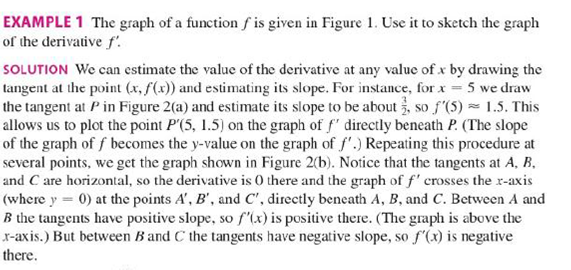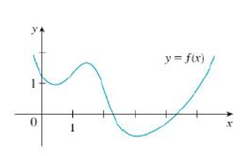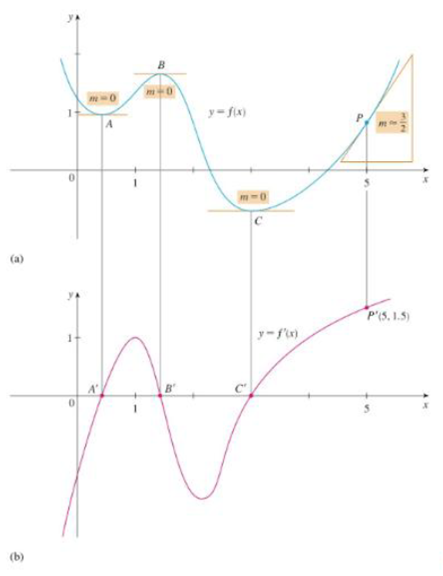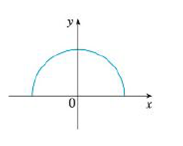Chapter 2.8, Problem 7E

Chapter
Section
Textbook Problem

Trace or copy the graph of the given function .f. (Assume that the axes have equal scales.) Then use the method of Example 1 to sketch the graph of f' below it.Example 1FIGURE 1FIGURE 2To determine

To sketch: The graph of f below the graph of f.

Explanation

From the given graph, it is observed that the graph of f contains the horizontal tangent at one point. Let the point be A.

Note that, the value of the derivative will be zero at the point where the function has the horizontal tangent.

Thus, the graph of f will be zero at the points A.

From the given graph, f has a vertical tangent line at the left end point. This implies that, the derivative function f has a vertical asymptote at that point. Thus, f is not differentiable at that point.

From the point A to left, the slope of the graph f is positive which implies that the derivative graph f must have a functional value in positive.

From the point A to right, the slope of the graph f is negative which implies that the derivative graph f must have a functional value in negative

Still sussing out bartleby?

Check out a sample textbook solution.

See a sample solution

The Solution to Your Study Problems

Bartleby provides explanations to thousands of textbook problems written by our experts, many with advanced degrees!

Get Started

In Exercises 35-38, find an equation of the line that has slope m and y-intercept b. 38. m=12;b=34

Applied Calculus for the Managerial, Life, and Social Sciences: A Brief Approach

Expand each expression in Exercises 122. (xx2)(x+x2)

Finite Mathematics and Applied Calculus (MindTap Course List)

Find the limit. 43. limni=1n1n(in)2

Single Variable Calculus: Early Transcendentals, Volume I

What is the defining characteristic of a repeated-measures or within-subjects research design?

Essentials of Statistics for The Behavioral Sciences (MindTap Course List)

Solve the following problems and reduce to lowest terms. 16.

Contemporary Mathematics for Business & Consumers

True or False: f(x) is symmetric about the origin if f(x) =f(x).

Study Guide for Stewart's Single Variable Calculus: Early Transcendentals, 8th

True or False: Let . Then f(x, y) is a joint probability function.

Study Guide for Stewart's Multivariable Calculus, 8th

Define the reliability of measurement and explain why and how it is measured.

Research Methods for the Behavioral Sciences (MindTap Course List)